## Least Squares Fitting--Exponential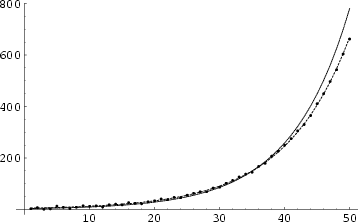To fit a functional form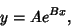(1)

take the Logarithm of both sides(2)

The best-fit values are then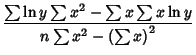(3)(4)

where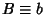and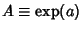.

This fit gives greater weights to smallvalues so, in order to weight the points equally, it is often better to minimize the function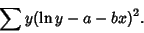(5)

Applying Least Squares Fitting gives(6)(7)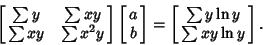(8)

Solving forand,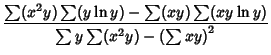(9)(10)

In the plot above, the short-dashed curve is the fit computed from (3) and (4) and the long-dashed curve is the fit computed from (9) and (10).

See also Least Squares Fitting, Least Squares Fitting--Logarithmic, Least Squares Fitting--Power Law